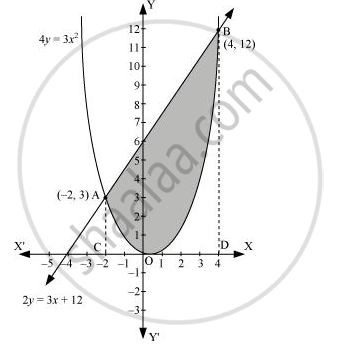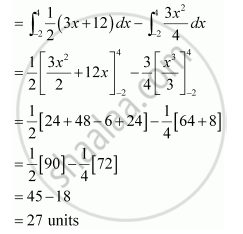Share
Notifications

View all notifications
Books Shortlist
Your shortlist is empty

# Find the Area Enclosed by the Parabola 4y = 3x2 and the Line 2y = 3x + 12 - CBSE (Commerce) Class 12 - Mathematics

Login
Create free account

Forgot password?

#### Question

Find the area enclosed by the parabola 4y = 3x2 and the line 2y = 3x + 12

#### Solution

The area enclosed between the parabola, 4y = 3x2, and the line, 2y = 3x + 12, is represented by the shaded area OBAO asThe points of intersection of the given curves are A (–2, 3) and (4, 12).

We draw AC and BD perpendicular to x-axis.

∴ Area OBAO = Area CDBA – (Area ODBO + Area OACO)Is there an error in this question or solution?

#### APPEARS IN

NCERT Solution for Mathematics Textbook for Class 12 (2018 to Current)
Chapter 8: Application of Integrals
Q: 7 | Page no. 375
Solution Find the Area Enclosed by the Parabola 4y = 3x2 and the Line 2y = 3x + 12 Concept: Area Under Simple Curves.
S